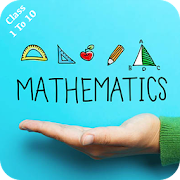# Mathematics For Class 1 To 10 Students - Lumos Educational App Store41
Price -FREE
\$0

#### DESCRIPTION:

Best app for Mathematics For Class 1 To 10 Students. Fun Math Tricks: 1. Addition 2. Subtraction 3. Multiplication table 4. Division 5. Multiply two digit number by 11 6. Square numbers ending in 5 7. Multiply by 5 8. Multiply by 9 9. Multiply by 4 10. Dividing by 5 11. Subtracting from 1000 12. Tough multiplication 13. Power of two 14. Adding numbers close to hundreds 15. Subtracting numbers close to hundreds 16. Multiply numbers between 11 and 19 17. Square numbers between 11 and 99 18. Multiply two digit numbers having same tens digit and ones digits add up to ten 19

#### OVERVIEW:

Mathematics For Class 1 To 10 Students is a free educational mobile app By Indian videos Apps.It helps students in grades 2 practice the following standards 2.OA.A.1,2.OA.B.2,2.NBT.A.1.

This page not only allows students and teachers download Mathematics For Class 1 To 10 Students but also find engaging Sample Questions, Videos, Pins, Worksheets, Books related to the following topics.

1. 2.OA.A.1 : Use addition and subtraction within 100 to solve one- and two-step word problems involving situations of adding to, taking from, putting together, taking apart, and comparing, with unknowns in all positions, e.g., by using drawings and equations with a symbol for the unknown number to represent the problem.

2. 2.OA.B.2 : Fluently add and subtract within 20 using mental strategies.

3. 2.NBT.A.1 : Understand that the three digits of a three-digit number represent amounts of hundreds, tens, and ones; e.g., 706 equals 7 hundreds, 0 tens, and 6 ones..

2

#### STANDARDS:

2.OA.A.1
2.OA.B.2
2.NBT.A.1

Developer: Indian videos Apps

Software Version: 1.2

Category: Education

### RELATED APPSEdSearch WebSearch